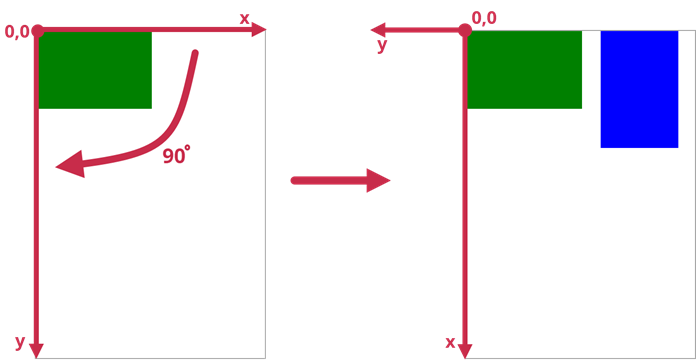.NET Framework 4.5.2+

# PdfGraphics.RotateTransform(Single) Method

Rotates the coordinate system clockwise to the specified angle relative to its origin.

Namespace: DevExpress.Pdf

Assembly: DevExpress.Pdf.v22.2.Drawing.dll

NuGet Package: DevExpress.Pdf.Drawing

## Declaration

``````public void RotateTransform(
float degree
)``````

## Parameters

Name Type Description
degree Single

Angle of rotation in degrees.

## Remarks

This method multiplies the transformation matrix of the PdfGraphics object by a rotation matrix. Elements of the rotation matrix are derived from the degree parameter.

The code sample below rotates the coordinate system and draws shapes in the initial and rotated coordinate systems.``````using DevExpress.Pdf;
using System.Drawing;
//...

using (PdfDocumentProcessor processor = new PdfDocumentProcessor())
{
processor.CreateEmptyDocument();
using (PdfGraphics graphics = processor.CreateGraphics())
{
// Draw a green rectangle.
using (var brush = new SolidBrush(Color.Green))
graphics.FillRectangle(brush, new RectangleF(0, 0, 300, 200));

// Rotate the coordinate system clockwise by 90 degrees.
graphics.RotateTransform(90);

// Draw a blue rectangle in the rotated coordinate system.
using (var brush = new SolidBrush(Color.Blue))
graphics.FillRectangle(brush, new RectangleF(0, -350, 300, -200));

// Add graphics content to the document page.